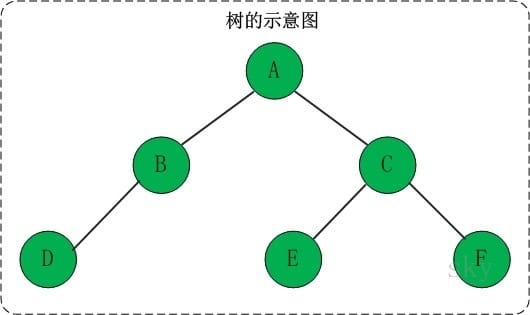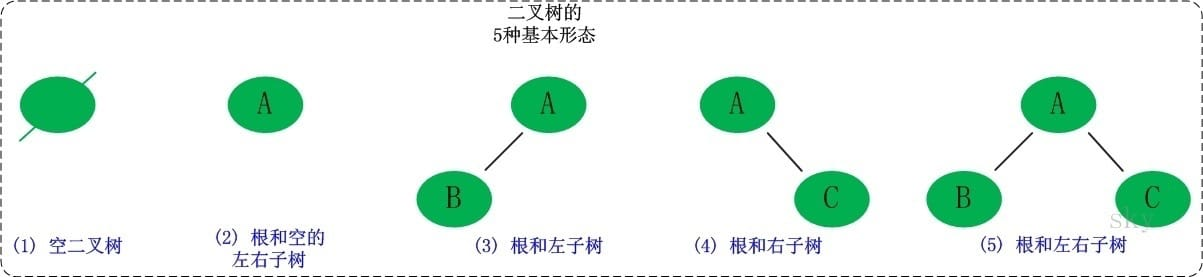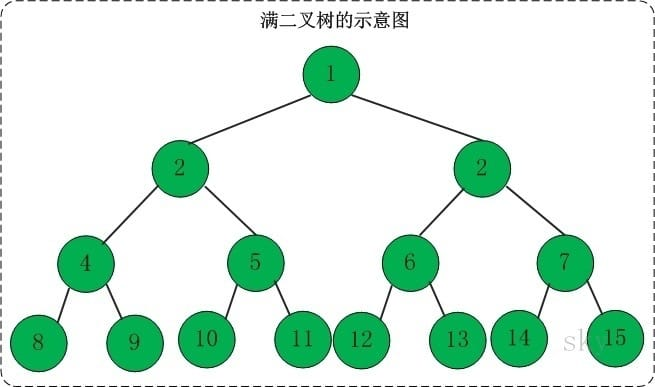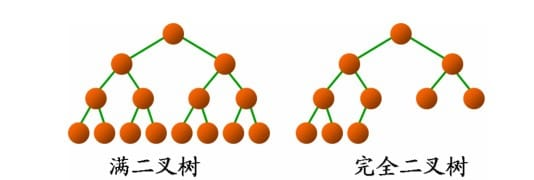## 一、二叉树的概念

### 1.1 树的基本概念• 每个节点有零个或多个子节点；
• 没有父节点的节点称为根节点；
• 每一个非根节点有且只有一个父节点；
• 除了根节点外，每个子节点可以分为多个不相交的子树。

• 结点的度：结点拥有的子树的数目。
• 叶子：度为零的结点。
• 分支结点：度不为零的结点。
• 树的度：树中结点的最大的度。
• 层次：根结点的层次为1，其余结点的层次等于该结点的双亲结点的层次加1。
• 树的高度：树中结点的最大层次。
• 无序树：如果树中结点的各子树之间的次序是不重要的，可以交换位置。
• 有序树：如果树中结点的各子树之间的次序是重要的, 不可以交换位置。
• 森林：0个或多个不相交的树组成。对森林加上一个根，森林即成为树；删去根，树即成为森林。
• ### 1.2 二叉树的定义

二叉树是每个节点最多有两个子树（不存在度大于2的结点）的树结构。二叉树的子树有左右之分，次序不能颠倒。它有5种基本形态：二叉树可以是空集；根可以有空的左子树或右子树；或者左右子树皆为空。### 1.3 二叉树的性质

#### 1.3.1 性质一

• 当$i=1$时，第$i$层的节点数目为$2^{i-1}=2^{0}=1$。因为第1层上只有一个根结点，所以命题成立。
• 假设当$i>1$，第$i$层的节点数目为$2^{i-1}$。这个是根据(01)推断出来的！
• 下面根据这个假设，推断出”第$(i+1)$层的节点数目为$2^{i}$”即可。由于二叉树的每个结点至多有两个孩子，故”第$(i+1)$层上的结点数目” 最多是 “第i层的结点数目的2倍”。即，第$(i+1)$层上的结点数目最大值$=2×2^{i-1}=2^{i}$。
• 故假设成立，原命题得证！

### 1.4 满二叉树和完全二叉树

#### 1.4.1 满二叉树1. 一棵树的深度为h，最大层数为k，深度与最大层数相同，k=h；
2. 叶子数为$2^h$
3. 第k层的结点数是：$2^{k-1}$；
4. 总结点数是$2^k-1$，且总节点数一定是奇数。

#### 1.4.2 完全二叉树## 二、二叉树的遍历

【提问】

1. 请分别写出并解释二叉树的先序、中序、后续遍历的递归与非递归版本
2. 给定二叉树的先序跟后序遍历，能不能将二叉树重建：不能，因为先序为父节点-左节点-右节点，后序为左节点-右节点-父节点，两者的拓扑序列是一样的，所以无法建立；如果换成一棵二叉搜索树的后续能不能建立：可以，因为只要将遍历结果排序就可以得到中序结果。

java

java

java

### 2.4 层序遍历